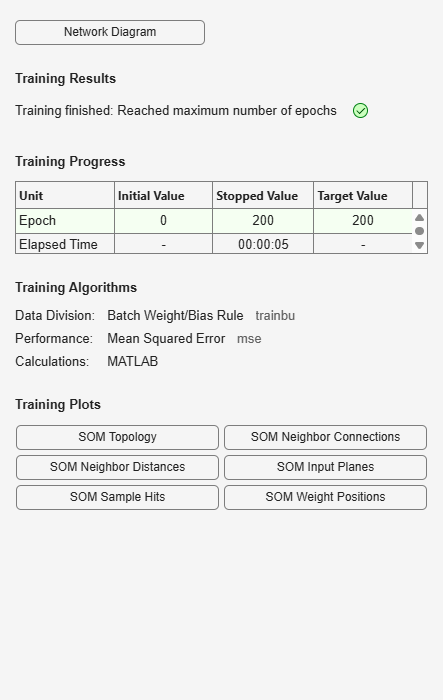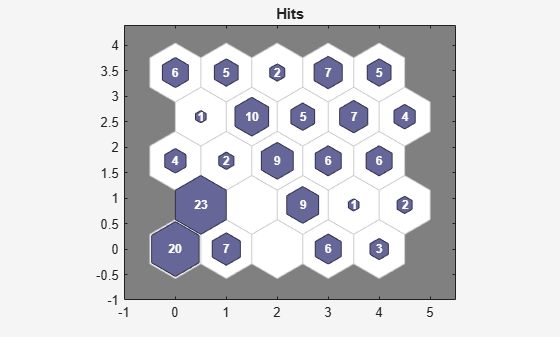# plotsomhits

Plot self-organizing map sample hits

## Syntax

```plotsomhits(net,inputs) ```

## Description

`plotsomhits(net,inputs)` plots a `SOM` layer, with each neuron showing the number of input vectors that it classifies. The relative number of vectors for each neuron is shown via the size of a colored patch.

This plot supports `SOM` networks with `hextop` and `gridtop` topologies, but not `tritop` or `randtop`.

## Examples

### Plot SOM Sample Hits

```x = iris_dataset; net = selforgmap([5 5]); net = train(net,x);````plotsomhits(net,x)`## Version History

Introduced in R2008a